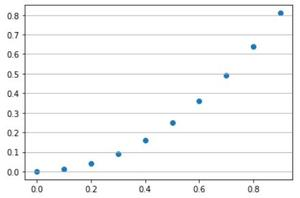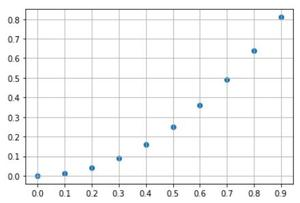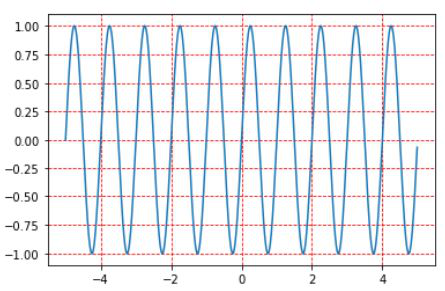Related Articles
How to add a grid on a figure in Matplotlib ?
• Difficulty Level : Basic
• Last Updated : 03 Jan, 2021

Matplotlib library is widely used for plotting graphs. In many graphs, we require to have grid to improve readability. Grids are created by using grid() function in pyplot sub library.

grid() : Configure the grid lines.

Syntax:

matplotlib.pyplot.grid(b=None, which=’major’, axis=’both’, **kwargs)

Parameters:

• b: bool or None, optional parameter for Whether to show the grid lines.
• which : {‘major’, ‘minor’, ‘both’}, optional. It specifies the grid lines to apply the changes on.
• axis : {‘both’, ‘x’, ‘y’}, optional. The axis to apply the changes on.
• **kwargs : Optional. Specify Line properties such as color, line style, line width, etc.

Steps:

• Plot a graph
• Specify grid using pyplot.grid() method.

Example 1:

## Python3

 `import` `matplotlib.pyplot as plt``import` `numpy`` ` `# Define x and y``x ``=` `numpy.arange(``0``, ``1``, ``0.1``)``y ``=` `numpy.power(x, ``2``)`` ` `# Plot graph``plt.scatter(x, y)`` ` `# Define grid with axis='y'``plt.grid(axis``=``'y'``)``plt.show()`` ` `# Define a figure``fig ``=` `plt.figure()``ax ``=` `fig.gca()`` ` `# Set labels on x and y axis of figure``ax.set_xticks(numpy.arange(``0``, ``1``, ``0.1``))``ax.set_yticks(numpy.arange(``0``, ``1``, ``0.1``))`` ` `# Plot the graph``ax.scatter(x, y)`` ` `# Specify default grid on figure``ax.grid()``ax.show()`

Output:Example 2:

## Python3

 `import` `matplotlib.pyplot as plt``import` `numpy as np`` ` `# Define x and y``x ``=` `np.arange(``-``5``, ``5``, ``0.01``)``y ``=` `np.sin(``2``*``np.pi``*``x)`` ` `# Plot line graph``plt.plot(x, y)`` ` `# Specify grid with line attributes``plt.grid(color``=``'r'``, linestyle``=``'--'``)`` ` `# Disply the plot``plt.show()`

Output:Attention geek! Strengthen your foundations with the Python Programming Foundation Course and learn the basics.

To begin with, your interview preparations Enhance your Data Structures concepts with the Python DS Course.

My Personal Notes arrow_drop_up